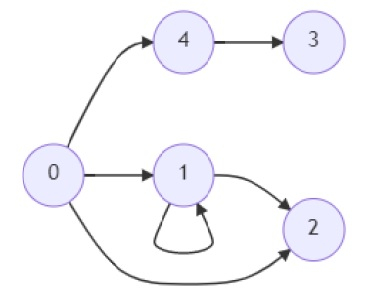# Program to check there is any common reachable node in a graph or not in Python

Suppose we have an edge list of a directed graph, there are n nodes and node names are 0 to n- 1. We also have two integer values a and b. We have to check whether there is any node c such that we can go from c to a and also c to b.

So, if the input is likeAnd a = 2, b = 3, then the output will be True, because here c = 0, so we have route from 0 to 2 and also 0 to 3.

To solve this, we will follow these steps −

• Define a function DFS() . This will take graph, node, visited
• if node is not visited, then
• mark node as visited
• for each x in graph[node], do
• DFS(graph, x, visited)
• From the main method, do the following −
• graph := generate adjacency list from the edge_list
• visited_a, visited_b := two empty sets
• DFS(graph, a, visited_a)
• DFS(graph, b, visited_b)
• ans := a new list from the intersection of visited_b and visited_a
• if ans is not empty, then
• return True
• return False

## Example

Let us see the following implementation to get better understanding −

def edge_list_to_graph(edges):
s = set()
for x, y in edges:
s = len(list(s))
graph = [[] for x in range(s)]
for x, y in edges:
graph[y].append(x)
return graph

def DFS(graph, node, visited):
if node not in visited:
for x in graph[node]:
DFS(graph, x, visited)

def solve(edges, a, b):
graph = edge_list_to_graph(edges)

visited_a, visited_b = set(), set()

DFS(graph, a, visited_a)
DFS(graph, b, visited_b)

ans = list(visited_a.intersection(visited_b))
if ans:
return True
return False

ed_list = [(0, 4),(4, 3),(1, 2),(0, 1),(0, 2),(1, 1)]
a = 2
b = 3
print(solve(ed_list, a, b))

## Input

[(0, 4),(4, 3),(1, 2),(0, 1),(0, 2),(1, 1)], 2, 3

## Output

True